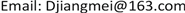﻿ 基于灰色模型的重庆市老龄化人口预测 Application of Grey Model in the Aging Population Forecast in Chongqing

Vol. 08  No. 07 ( 2019 ), Article ID: 31436 , 5 pages
10.12677/AAM.2019.87145

Application of Grey Model in the Aging Population Forecast in Chongqing

Jiangmei Duan

Department of Mathematics and Statistics, Zhaotong University, Zhaotong YunnanReceived: July 4th, 2019; accepted: July 19th, 2019; published: July 26th, 2019ABSTRACT

According to the statistical yearbook data of Chongqing in 2008-2017, the grey forecasting model is used to predict the aging population in Chongqing in the next decade. It is predicted that the population aged 65 and over will reach 5.1103 million in 2027, and the aging coefficient will increase year by year. Therefore, the degree of aging continues to increase.

Keywords:Grey Model, Aging Population, Aging Coefficient1. 引言

2. 灰色GM(1,1)模型的建立

2.1. 灰色GM(1,1)模型的建立

${X}^{\left(0\right)}=\left\{{X}^{\left(0\right)}\left(1\right),{X}^{\left(0\right)}\left(2\right),\cdots ,{X}^{\left(0\right)}\left(n\right)\right\}$ 是原始数据序列，对 ${X}^{\left(0\right)}$ 进行一次累加生成，得到累加生成序列 ${X}^{\left(1\right)}=\left\{{X}^{\left(1\right)}\left(1\right),{X}^{\left(1\right)}\left(2\right),\cdots ,{X}^{\left(1\right)}\left(n\right)\right\}$ ，其中 ${X}^{\left(1\right)}\left(t\right)=\underset{i=1}{\overset{t}{\sum }}{X}^{\left(0\right)}\left(i\right)$$t=1,2,\cdots ,n$

$\left\{\begin{array}{l}\frac{\text{d}{X}^{\left(1\right)}\left(t\right)}{\text{d}t}+a{X}^{\left(1\right)}\left(t\right)=u\\ {X}^{\left(1\right)}\left(1\right)={X}^{\left(0\right)}\left(1\right)\end{array}$

$\stackrel{^}{a}={\left[a,u\right]}^{\text{T}}={\left({B}^{\text{T}}B\right)}^{-1}{B}^{\text{T}}Y$

${\stackrel{^}{X}}^{\left(1\right)}\left(t+1\right)=\left({X}^{\left(0\right)}\left(1\right)-\frac{u}{a}\right){\text{e}}^{-at}+\frac{u}{a}$$t=1,2,\cdots ,n$

${\stackrel{^}{X}}^{\left(0\right)}\left(t+1\right)={\stackrel{^}{X}}^{\left(1\right)}\left(t+1\right)-{\stackrel{^}{X}}^{\left(1\right)}\left(t\right)$$t=1,2,\cdots ,n$

2.2. 模型精度的检验 

1) 残差检验

2) 后验差检验

3. 利用灰色模型预测重庆市老龄化人口  

3.1. 研究区概况

3.2. 数据收集Table 2. Statistical table of total population, population aged 65 and above and aging coefficient of Chongqing from 2008 to 2017

3.3. 重庆市老龄化人口预测Table 3. Grey G M ( 1 , 1 ) model prediction value and relative error (ten thousand people)Table 4. Prediction of population aged 65 and above and aging coefficient in Chongqing from 2018 to 2027

Application of Grey Model in the Aging Population Forecast in Chongqing[J]. 应用数学进展, 2019, 08(07): 1251-1255. https://doi.org/10.12677/AAM.2019.87145

1. 1. 陈威杰, 吕盛鸽. 四川省人口老龄化系数中长期预测[J]. 统计与决策, 2013(1): 125-128.

2. 2. 石端银, 李文宇, 蔡吉花, 等. 改进的灰色增量模型及其在哈市人口预测中的应用[J]. 数学的实践与认识, 2013, 43 (10): 71-76.

3. 3. 刘卜铭, 徐跃通. 基于灰色GM(1,1)模型的山东省人口预测与可持续发展研究[J]. 鲁东大学学报(自然科学版), 2015, 31(3): 254-259.

4. 4. 蒋诗泉. 基于灰色理论的人口老龄化发展趋势及其影响因素研究[J]. 华东师范大学学报(哲学社会科学版), 2014, 46(3): 135-139.

5. 5. 郑丽, 孜比布拉•司马义, 颉渊, 等. 基于两种灰色模型的乌鲁木齐市人口预测及其人口问题的探讨[J]. 干旱区资源与环境, 2016, 30(11): 77-84.